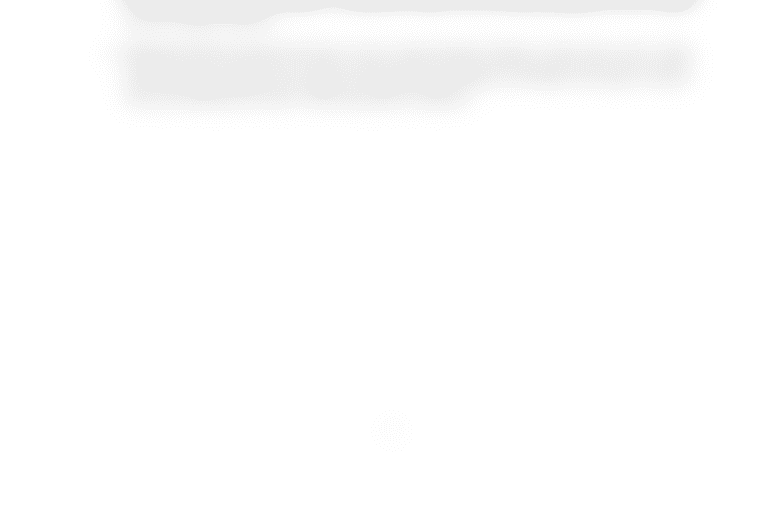Class Notes (1,100,000)
CA (620,000)
UW (20,000)
MATH (2,000)
MATH135 (300)
Lecture 3

# MATH135 Lecture 3: Exercises - Linear Diophantine Equations

Department
Mathematics
Course Code
MATH135
Professor
Roxane Itier Itier
Lecture
3

This preview shows half of the first page. to view the full 1 pages of the document.MATH 135, Winter 2015
Exercises: Linear Diophantine Equations
1. Decide whether the following linear Diophantine equations admit solutions (without solving
them).
(a) 4x7y 100
(b) 42x56y21
(c) 6x22y18
2. Find a general solution to the following linear Diophantine equations.
(a) 6x4y60
(b) 27x78y12
(c) 81x24y6
3. For each equation in the previous question, ﬁnd all positive solutions, if any exist.
4. When Mr. Smith cashed a cheque for xdollars and ycents, he received instead ydollars and
xcents, and found that he had two cents more than twice the proper amount. For how much
was the cheque written?
5. (Hundred Fowls Problem) Roosters costs 5 qian (ancient Chinese coins) each, hens 3 qian
each and three chickens cost 1 qian. If 100 fowls are bought for 100 qian, how many roosters,
hens and chickens are there? Find all positive solutions.
1
###### You're Reading a Preview

Unlock to view full version